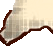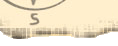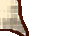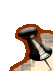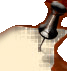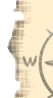Rhode Island Mineral Hunters Feature Page
HomePhoto Album PageMeetingsNewsletterField TripsFeature PageAbout UsContact USLinks & ShowsAnnual Show

How Do You Know If A Geode Is Hollow Without Opening It?

Everyone knows what a geode is, a spherical rock with a hidden hollow inside usually encrusted with some type of crystals. Here’s the caveat though, how do we know if the cavity inside is large or small, that it has lots crystals of just few. Most experienced geode breakers knew a simple "rule of thumb," if one geode of other geodes of the same size weighs less than the others then the lighter one probably had a larger hollow center, thus able to hold more crystals.

Well there is a more definitive and mathematical way for calculating whether one geode is more hollow than another. This way is based on the density of quartz. Forgoing all the deep math to calculate the density of quartz, we’ll just plug in the numbers and put forth this simple formula.

Weight (if solid) in pounds (lb) = 0.401 x r3 where r is the outside radius of the geode in inches. As you remember from high school geometry r is one-half the diameter and the little 3 above and to the right of the r is an exponent which means you multiply r by itself 3 times! (Didn’t you love geometry and algebra?). So if a geode weighs less than it should by this formula, then it as a hole inside. The greater the weight difference the bigger the hole inside should be!

So here it is:

Weight (if solid) = 0.401 x r3

So lets’ try it out. Lets’ take a geode with a diameter of 8 inches and plug it into our formula.

First if the diameter is 8 inches then the radius (r) is 8 divided by 2 (8/2) or 4 inches.

Now we can plug our numbers into the formula.

Weight = 0.401 x 43 = 0.401 x (4 x 4 x 4) = 0.401 x 64 = 24.664lbs

Any geode weighing considerably less would have a greater hollow inside.

So the next time you step up to the geode seller have your scale and calculator ready and get the best geode money can buy.

Good Luck.

Somewhere out there the great hollow geode filled with crystals is waiting for you.

GEODE WEIGHT CHART FOR QUARTZ

(solid wt. = 0.401 x r3)

 GEODE DIAMETER WEIGHT IF SOLID 3.00 in 1.35 lbs 3.50 in 2.15 lbs 4.00 in 3.21 lbs 4.50 in 4.57 lbs 5.00 6.27 lbs 5.50 in 8.34 lbs 6.00 in 10.83 lbs 6.50 in 13.77 lbs 7.00 in 17.20lbs 7.50 in 21.15 lbs 8.00 in 25.66 lbs 8.50 in 30.80 lbs 9.00 in 36.50 lbs 9.50 in 43.00 lbs 10.00 in 50.12 lbs

Special Feature